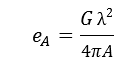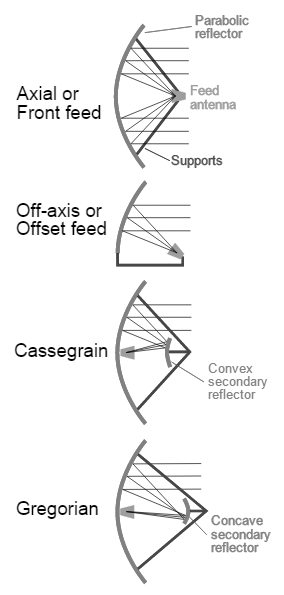# Antenna Efficiency Calculator

This CalcTown calculator calculates the efficiency of a parabolic antenna given its aperture, gain and wavelength of radio waves.

m2

#### ResultClick here to view image

Where,

A = aperture area of the parabolic antenna

λ = Wavelength of radio waves

G = Gain of the parabolic antenna

eA = Efficiency of antenna aperture